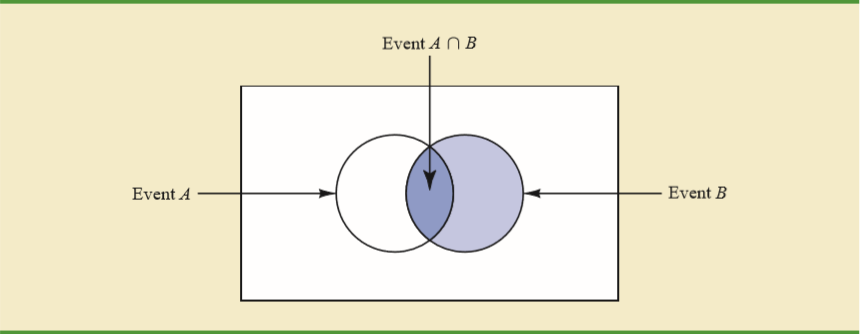# Conditional Probability §

Conditional probability is the degree of belief in a proposition given some evidence that has already been revealed.

Conditional Probability

is the probability of given (i.e. given that is true).

Visualization:Visualize as the dark blue area (intersection area) over the light blue area ().

Other way to arrange, multiplying events:

This is basically the Chain Rule for probability! Idea from here

Other useful equation: